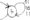# Logic design July 2008

Note : Answer any FIVE full questions, choosing at least two from each part.

PART-A

1 a. What are universal gates? Implement the following function using universal gates only ((A + B)c)d

b.  Simplify the following using K – map F(A, B, C, D) ~ ABC + AD + BD + CD + AC + a5 .

c.   What are the drawbacks of k-map? Simplify the following expression using Quine – Me Clusky Method. F(A, B, C, D) = S(l,2,8,9,l 0,12,13,14).

2    a. Show that using a 3 – to – 8 decoder and multi -input OR gate. The following Boolean expressions can be realized. F1(A,B,C) = £m(0,4,6), F2(A,B,C)= £111(0,5), F3,(A,B,C)= Em(l,2,3,7).

b. Design Decimal – to – BCD encoder?

c.   What are the different types of PLD’s and implement the 7 – segment decoder using PLA?

d.   Write a verilog code for 4 : 1 multiplexer using case statement.

3 a. i) Perform 8 – bit addition of the decimal numbers – 28 and + 15 in 2’s complement.

ii) Perform 8 – bit subtraction of the decimal numbers – 28 and + 65 in 2’s complement.

b.  i) Find the binary addition of (7510)io and (538)io using 16 – bit numbers

ii) Find the binary subtraction of (2QO)io and (125)io using 8 – bit numbers.

c.   Explain the binary Adder – subtracted circuit with an example.

4  a. What is Schmitt trigger? Explain Schmitt trigger transfer characteristic.

b. Explain the different types of flip fops along with their truth table. Also explain the race-around condition in a flip flop,

c. Differentiate between combinational circuit and sequential circuit.

PART-B

5    a. Explain a 4 -bit serial input shift registers in detail and give its timing diagram.

b.   Design a mod – 5 synchronous up counter using JK flip flop.

6 a. Explain Moore model with state synthesis table and also obtain the circuit diagram for Moore model.

b.   Design an asynchronous sequential logic circuit for state transition diagram shown in Fig.Q 6(b).

7 a. What is a binary ladder? Explain the binary ladder with a digital input of 1000.

b.   Explain a 2 – bit simultaneous A/D converter.

8 a. With a circuit diagram, explain the operation of the CMOS NAND gate.

b.   Explain a 2 – input NAND gate TTL with Totem – pole output with a neat